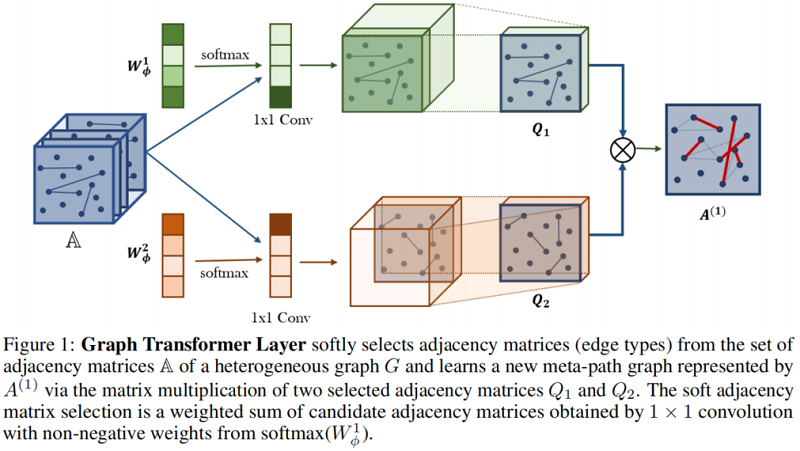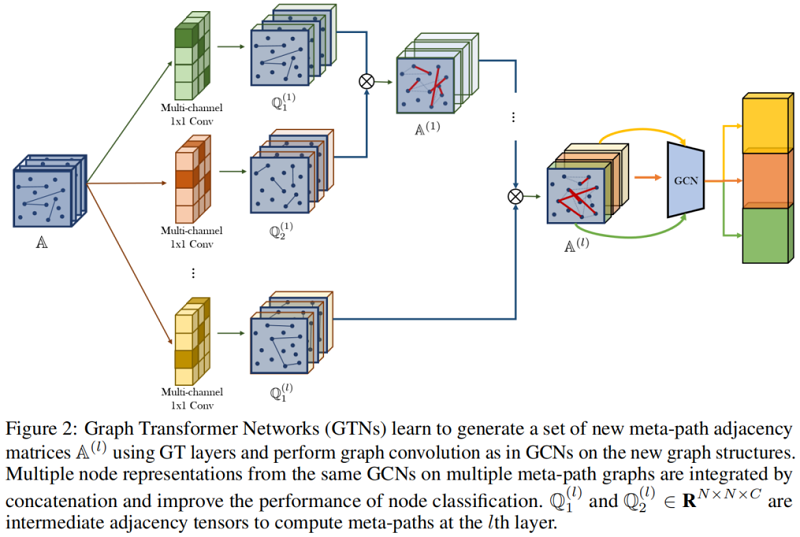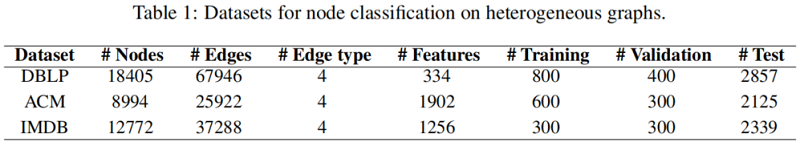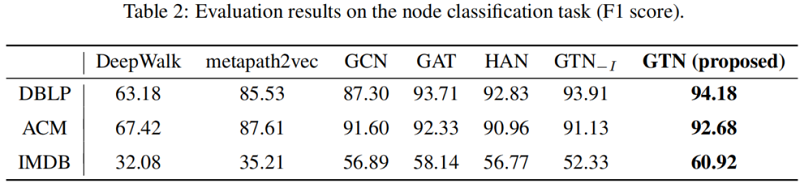# 论文解读（GTN）《Graph Transformer Networks》

2022-05-09 16:13 190 查看

# 1 Introduction

GNNs 基于的理论假设：

• [li]图结构固定（fixed）
• 同质性（homogeneous）
[/li]

# 2 Method

## 2.1 Preliminaries

GTN 框架的一个输入是具有不同类型的节点和边的多图结构。设 $T^{v}$ 和 $T^{e}$ 分别是节点类型和边类型的集合。输入图可以看作是一个异质图 $G=(V, E)$ ，其中 $V$ 是节点集， $E$ 是可观察到的边集，还有一个节点类型映射函数 $f_{v}: V \rightarrow T^{v}$ ，一个边类型映射函数 $f_{e}: E \rightarrow \mathcal{T}^{e}$ 。每个节点 $v_{i} \in V$ 都有一个节点类型，即 $f_{v}\left(v_{i}\right) \in T^{v}$。同样地，对于 $e_{i j} \in E$ ， $f_{e}\left(e_{i j}\right) \in T^{e}$ 。当 $\left|T^{e}\right|=1$ 和 $\left|T^{v}\right|=1$ 时，它就成为了一个标准图。在本文中，考虑 $\left|T^{e}\right|>1$ 的情况。设 $N$ 表示节点数，即 $|V|$ 。异质图可以用 一组邻接矩阵 $\left\{A_{k}\right\}_{k=1}^{K}$ 表示， 其中 $K=\left|T^{e}\right|$ ， $A_{k} \in R^{N \times N}$ 是一个邻接矩阵，当从 $j$ 到 $i$ 有 $k$ 类型的边时， $A_{k}[i, j]$ 非零，可被简写成一个张量 $A \in R^{N \times N \times K}$ 。特征矩阵 $X \in R^{N \times D}$ ，意味着每个节点的输入特征为 $D$ 维。

Meta-Path

由 $p$ 表示，是异质图 $G$ 上由异构的边连接的一条路径，即 $v_{1} \stackrel{t_{1}}{\longrightarrow} v_{2} \stackrel{t_{2}}{\longrightarrow} \ldots \stackrel{t_{l}}{\longrightarrow} v_{l+1}$ ，其中 $t_{l} \in T^{e}$ 表示元路径中的一条 $l$ 类型的边。它定义了节点 $v_{1}$ 和 $v_{l+1}$ 之间的一个复合关系 $R=t_{1} \circ t_{2} \ldots \circ t_{l}$ ，其中 $R_{1} \circ R_{2}$ 表示关系 $R_{1}$ 和 $R_{2}$ 的组成。给定复合关系 $R$ 或边类型序列 $\left(t_{1}, t_{2}, \ldots, t_{l}\right)$ ，元路径 $P$ 的邻接矩阵 $A_{P}$ 是通过邻接矩阵的乘法得到的：

$A_{\mathcal{P}}=A_{t_{l}} \ldots A_{t_{2}} A_{t_{1}} \quad\quad\quad(1)$

元路径的概念包含了多跳连接，在我们的框架中，新的图结构由邻接矩阵表示。例如，元路径 Author-Paper-Conference (APC) 可以表示为  $A \stackrel{A P}{\longrightarrow} P \stackrel{P C}{\longrightarrow} C$，通过 $A_{A P}$ 和 $A_{P C}$ 的多重生成邻接矩阵 $A_{A P C}$。

Graph Convolutional network (GCN)

标准GCN ：

$H^{(l+1)}=\sigma\left(\tilde{D}^{-\frac{1}{2}} \tilde{A} \tilde{D}^{-\frac{1}{2}} H^{(l)} W^{(l)}\right) \quad\quad\quad(2)$

其他形式：

$H^{(l+1)}=\sigma\left(\tilde{D}^{-1} \tilde{A} H^{(l)} W^{(l)}\right)$

## 2.2 Meta-Path Generation

Fig. 1 中 Graph Transformer (GT) 层新元路径图生成：Graph Transformer (GT) Layer 有两个组成部分：

• [li]首先，GT 层从候选邻接矩阵 $A$ 中选择两个图结构 $Q_{1}$ 和 $Q_{2}$；
• 其次，它通过组成两个关系(即两个邻接矩阵的矩阵乘法，$Q_{1} Q_{2}$ )来学习一个新的图结构；
[/li]

邻接矩阵 $Q$ 的选择方式如下：

$Q=F\left(\mathbb{A} ; W_{\phi}\right)=\phi\left(\mathbb{A} ; \operatorname{softmax}\left(W_{\phi}\right)\right) \quad\quad\quad(3)$

其中， $\phi$ 是卷积层，$W_{\phi} \in \boldsymbol{R}^{1 \times 1 \times K}$ 是 $\phi$ 的参数。即 $Q$ 是将 $\mathbb{A}$  和权重参数 $W_{\phi}$ 送去卷积层卷积得到的。

每一个 $Q_{i}$ 可以表示成：

$\sum_{t_{l} \in T^{e}} \alpha_{t_{l}}^{(l)} A_{t_{l}}$

其中， $T^{e}$ 是边的类型集合， $\alpha_{t l}^{(l)}$ 是第 $l$ 种边类型 $t_{l}$ 在第 $l$ 层的权重。

以 Fig.1 为例：

$T^{e}$ 有 $4$ 个 $\left\{t_{1}, t_{2}, t_{3}, t_{4}\right\}$ ，即对应 $4$ 层矩阵: $\left\{A_{1}, A_{2}, A_{3}, A_{4}\right\}$， $W_{\phi}=\left\{\alpha_{1}, \alpha_{2}, \alpha_{3}, \alpha_{4}\right\}$ 。

得到 $Q_{1}$ 和 $Q_{2}$ 后，通过两个邻接矩阵的矩阵乘法 $Q_{1} Q_{2}$ 得到新的元路径邻接矩阵。为了提高数值稳定性，通过该矩阵的度矩阵将归一化， 即 $A^{(l)}=D^{-1} Q_{1} Q_{2}$ 。

现在，我们需要检查GTN是否可以学习关于边类型和路径长度的任意元路径。任意长度 $l$ 元路径的邻接矩阵可以表示为:

$A_{P}=\left(\sum_{t_{1} \in \mathcal{T}^{e}} \alpha_{t_{1}}^{(1)} A_{t_{1}}\right)\left(\sum_{t_{2} \in \mathcal{T}^{e}} \alpha_{t_{2}}^{(2)} A_{t_{2}}\right) \ldots\left(\sum_{t_{l} \in \mathcal{T}^{e}} \alpha_{t_{l}}^{(l)} A_{t_{l}}\right) \quad\quad\quad(4)$

其中  $A_{P}$  表示元路径的邻接矩阵， $T^{e}$  是边的类型集合，  $\alpha_{t_{l}}^{(l)}$  是第  $l$  个 GT 层上边类型  $t_{l}$  的权重。当  $\alpha$  不是 one-hot 向量时，  $A_{P}$  可以看作是所有长度为  $l$  元路径邻接矩阵的加权和。因此，一个  $l$  个GT层的堆栈可以学习任意长度的  $l$  元路径图结构，如 Fig. 2 所示。这种结构的一个问 题是，添加 GT 层总是增加元路径的长度，而不允许使用原始的边。在一些应用中，长元路径和短元路径都很重要。为了学习包含原始边 的短元路径和长元路径 (如果全部按照图中的生成，则每一条元路径都是基于原始边所生成的，也就忽略了原始边的本身的特征)，我们 在  $\mathbb{A}$  中加入单位矩阵  $I$  ，即  $A_{0}=I$  。这个技巧允许 GTN 学习任何长度的元路径，当  $l$  个GT层堆叠时，元路径的长度可达  $l+1$  。## 2.3 Graph Transformer Networks

为了同时生成多种类型的元路径，Fig. 1 中  $1 \times 1$  卷积的输出通道设置为  $C$  。然后，GT 层产生一组元路径，中间邻接矩阵  $Q_{1}$  和  $Q_{2}$  成为邻接 张量  $Q_{1}$  和  $Q_{2} \in \boldsymbol{R}^{N \times N \times C}$  ，如 Fig.2 所示。通过多个不同的图结构学习不同的节点表示是有益的。在  $l$  个 GT 层堆叠之后，将 GCN 应用于元 路径张量  $\mathbb{A}^{(l)} \in \boldsymbol{R}^{N \times N \times C}$  的每个通道，并将多个节点的表示连接起来:

$Z=\|_{i=1}^{C} \sigma\left(\tilde{D}_{i}^{-1} \tilde{A}_{i}^{(l)} X W\right) \quad\quad\quad(5)$

其中， $\|$ 为连接运算符， $C$ 表示通道数， $\widetilde{A}_{i}^{(l)}=A_{i}^{(l)}+I$ 表示张量 $\mathbb{A}^{(l)}$ 的第 $l$ 个通道的邻接矩阵， $\widetilde{D}_{i}$ 是 $\widetilde{A}_{i}^{(l)}$ 的度矩阵， $W \in R^{d \times d}$ 是跨通道共享的可训练权重矩阵， $X \in \boldsymbol{R}^{N \times d}$ 是一个特征矩阵。

$Z$ 包含来自 $C$ 个不同的元路径图的节点表示，该图具有可变性、长度最多为 $l+1$ 。它用于顶部的节点分类。损失函数是具有真实标签的节点上的标准交叉熵。

# 3 Experiments# 4 Conclusion

我们提出了用于学习节点表示的图变换网络。我们的方法将异构图转换为多个新的图，这些图定义的任意边类型和任意长度小于图变换层数的元路径，同时通过对学习的元路径图的卷积学习节点表示。学习到的图结构导致更有效的节点表示，在所有三个基准节点分类的异构图上产生最先进的性能。由于我们的图形转换层可以与现有的gnn结合，我们相信我们的框架为gnn开辟了新的方法，可以自己优化图结构，从而根据数据和任务操作卷积，而无需任何人工努力。有趣的未来方向包括研究GT层与不同类别的gnn结合，而不是GCNs的疗效。此外，由于一些异构图数据集最近被研究用于其他网络分析任务，如链路预测[36,41]和图分类[17,24]，将我们的gtn应用于其他任务可能是有趣的未来方向。